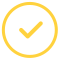effortlessly.

# What is the Tangent Line?

It refers to a line touching a curve at a particular point. The meeting point of the line and the curve is the point of tangency. Therefore with this calculator, you can be able to calculate the slope-intercept.

## Tangent Line Calculator For You To Use

It is an online tool that helps you find the tangent line to the implicit, explicit, parametric, and polar curve at a given point. On top of that, it can act as a horizontal tangent calculator by helping you find the vertical and horizontal tangent lines as well.

### The Equation of Tangent Line: Calculator Formula

We use the following variables to find an equation of the tangent line to the curve at the given point:

• The slope of the tangent line
• Where the tangent line is passing through on the curve

Now, to find the tangent line, use the formula below:

(y - y1) = m (x - x1)

To understand this concept, let us look at an example:

Find the tangent equation to the parabola y2 = 10x at the point (2, -4):

Solution: y2 = 10x

Differentiate with respect to "x",

2y (dy/dx) = 10(1)

m = dy/dx = 10/2y ==> 4/y

Slope at the point (2, -4)

m = 4/ (-4) ==> -1

Equation of Tangent:

(y - y1) = m(x - x1)

(y - (-4)) = (-1) (x - 2)

y + 4 = -x + 2

x + y + 4 - 2 = 0

x + y + 2 = 0

When using the slope of tangent line calculator, the slope-intercept formula for a line is found by the formula below:

y = mx + b

Where

• m stands for the slope of the line
• b is the y-intercept

For instance, when you enter the curve, y= 4x^2-4x+1 at x=1, in our tangent line finder, the result will be as follows:

y= 4x2-4x+1 at x=1

Result=4

Equation of the tangent line: y= 4x-3

Students can then plot the graph or use our readily available math help.

### How To Use The Tangent Line Calculator

The calculator has the average student in mind. You do not need to therefore worry about any technicalities when using it. Below are the simple steps to follow:

• First, enter the curve equation in the first and second input fields (x and y values)
• After entering the values correctly, click on the “Calculate” button to get the results
• You will find the slope value and the equation of the tangent line in a new window

Our tangent line calculator also gives you the expected plot graph. It will ensure that you get everything correctly for top results.

### We Can Help With Your Math Homework

If you feel that the calculator answers are not sufficient, just say "do my math for me" - our professional math solvers are here. We have math gurus who are Masters and Ph.D. holders with years of experience handling math problems.

Get your tangent line problem solved now!

Let’s make your assignment go away.Awesome. Now you'll never miss out.